# Addition and Subtraction of Integers

Go back to  'Integers'

## Introduction to Addition and Subtraction of Integers

If you are comfortable with visualising the addition and subtraction of whole numbers on the number line, the same concept can be easily extended to integers.

## The big idea behind addition and subtraction of integers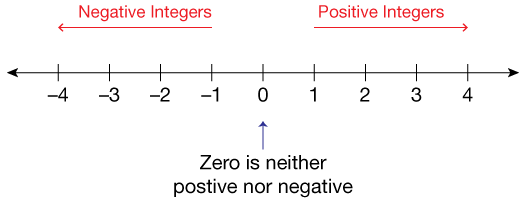How integers function is better understood with the help of the number line.

Numbers to the right are positive numbers, numbers to the left are negative numbers, and zero is neither positive nor negative.
As understood, the basic principles of addition are repeatedly adding into a particular group and subtracting is nothing but removing from a group. Operations on integers work similarly, but with a twist

### The rules of the addition of integers

We begin from 0, working our way towards the left of the number line and stopping at 8. An additional 9 has to be added since the 9 has a negative sign, we move towards the left of the number line. From 8 we move 9 steps towards the left, landing you at 17.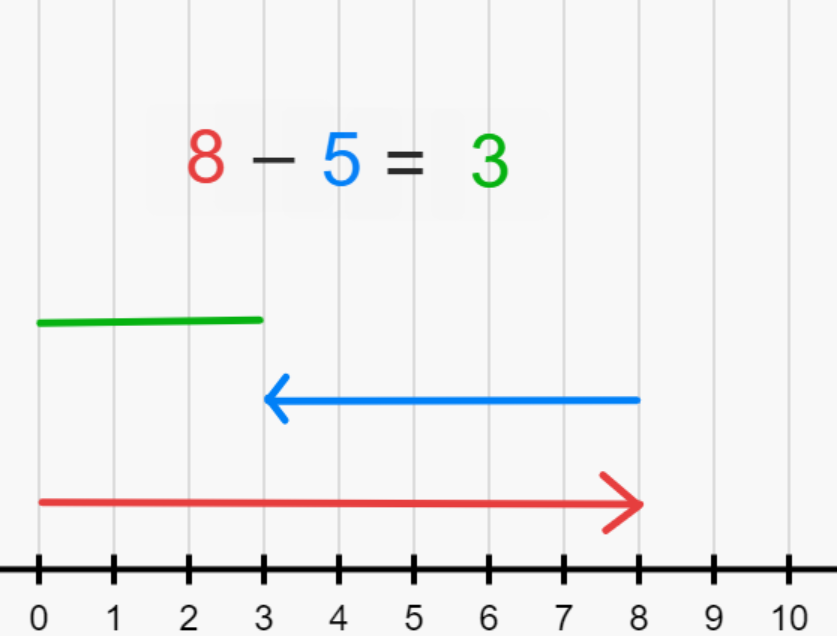If both numbers have the same sign, add both numbers and include the same sign as both.

Example:

1. 4 + 8 = 12
Using the number line, we start at zero and move to 4. The equation calls for another additional number 8. From 4, move 8 steps forwards towards the right of the number line, leaving you at 12.
2. –7 + (–10) = –17
Using the number line, we begin at 0 and move to the left and stop at –7. Since the equation calls for an additional –10, we move another 10 steps towards the left, leaving you at –17.

If both numbers have different signs, add or subtract the number and include the sign of the largest absolute integer.

Example:

1. 10 + (– 4)
a. 10 – 4
b. 6
We begin at 0, moving to 10 towards the right of the number line. The equation then asks you to add a –4. But a negative sign means you have to travel to the left of the number line, making you move 4 spaces towards the left from 10. Landing you at 6.
2. –8 + –9
a. 8 – 9 = –17
b. –9 is larger than 5

### The rules of subtraction of integers

If both numbers have positive signs, the difference will be positive.

Example:

1. 20 – 18 = 2
We begin from 0, working our way towards the right of the number line and stopping at 20.
Since 18 has a negative sign, we move back towards the left of the number line. From 20 we move 18 steps towards the left, landing you at 2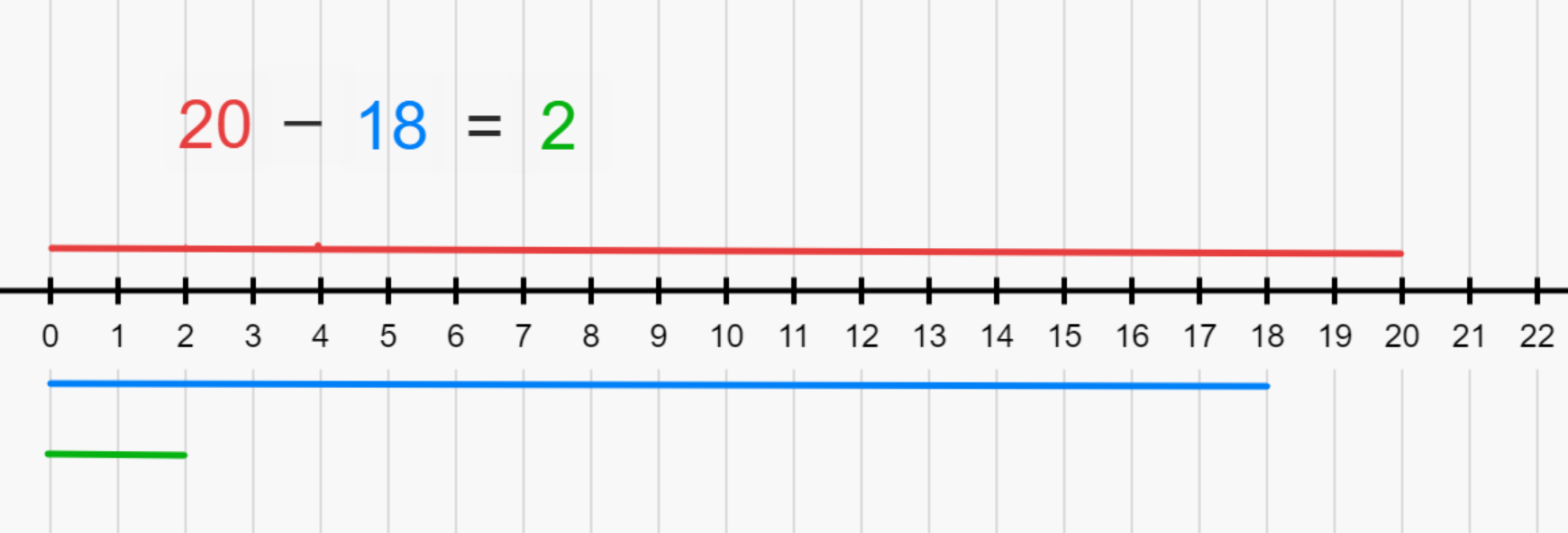If both numbers have a negative sign, subtract the two numbers and include the sign of the largest absolute value.

Example:

1. –10 – ( –15)
a. –10 + 15 according to the rules of multiplication 2 negatives make a positive.
b. 15
15 is larger than 10

We begin from 0, working our way towards the left of the number line and stopping at -10. Since two negatives make a positive, making – (–15) = 15, we move forwards towards the right of the number line. From –10 we move 15 steps towards the right, landing you at 5.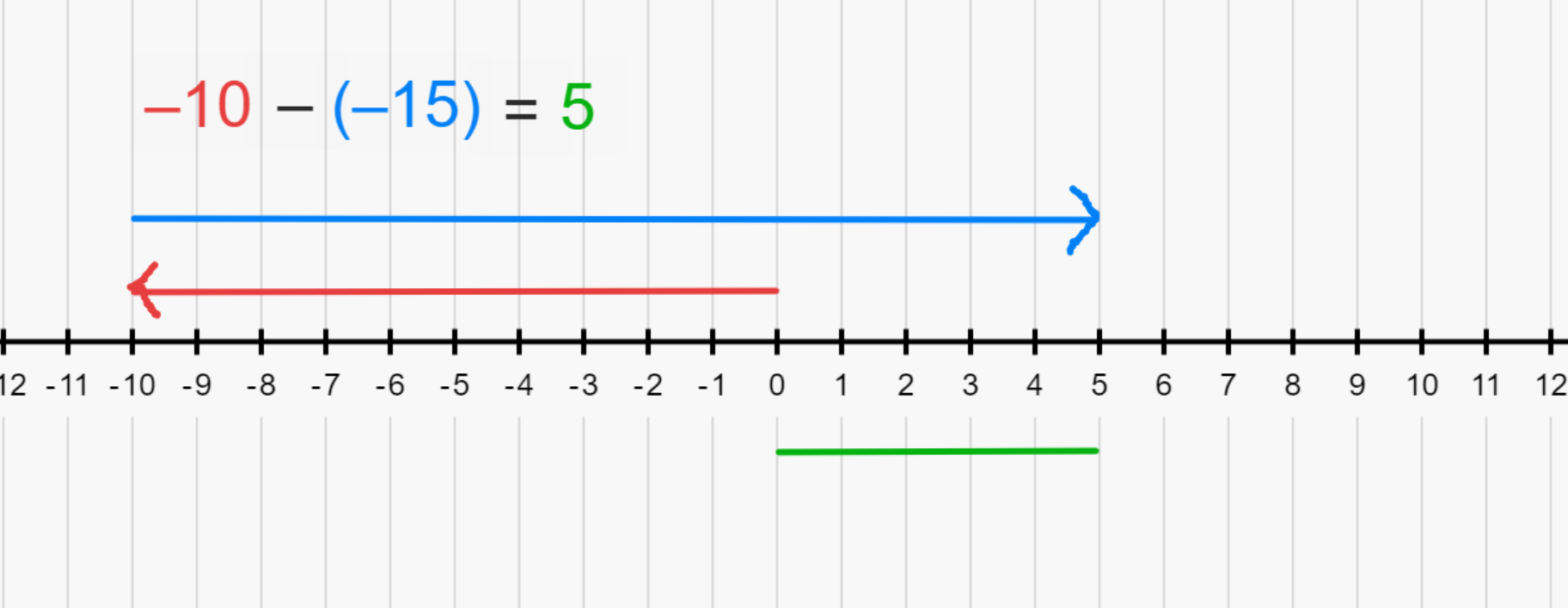If both numbers have different signs, subtract the two and denote the difference with the sign of the largest absolute value.

Example:

1. 18 – 5 = 13
18 is larger than 3 and has a positive value
2. –6 – 3 = –9
We begin from 0, working our way towards the left of the number line and stopping at –6. Since 3 has to be subtracted, we move 3 steps towards the left from –6. Landing you at –9.

## Fun Facts about Integers and Signs

One thing that children struggle with it is signs that precede integers, specifically the negative sign. Here is a very simple algorithm to help visualise and internalise the sign changes and how that affects the direction of a number on the number line:
Let’s the number 10 for our particular example (keep in mind this rule will apply to all integers):

10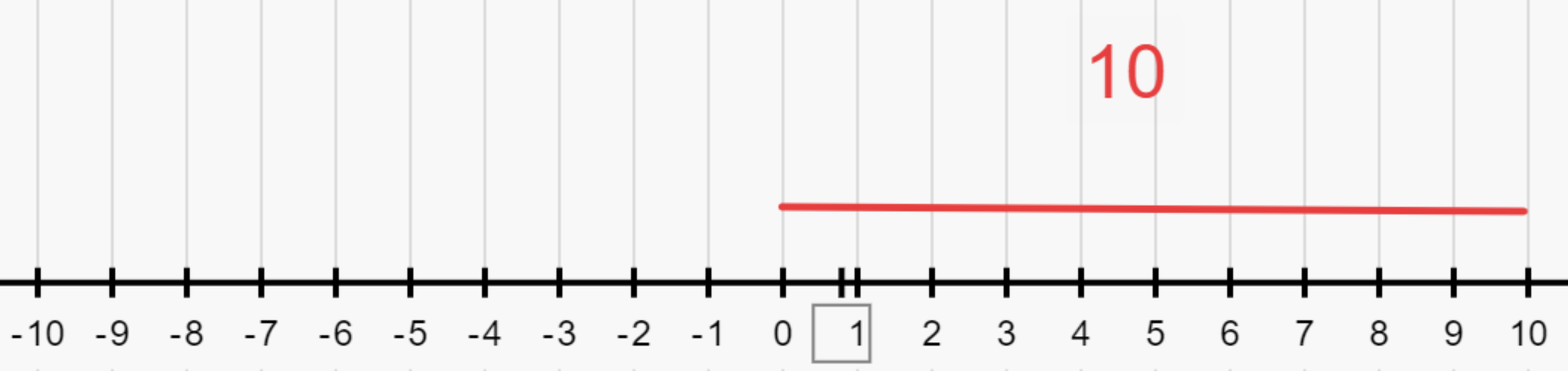–10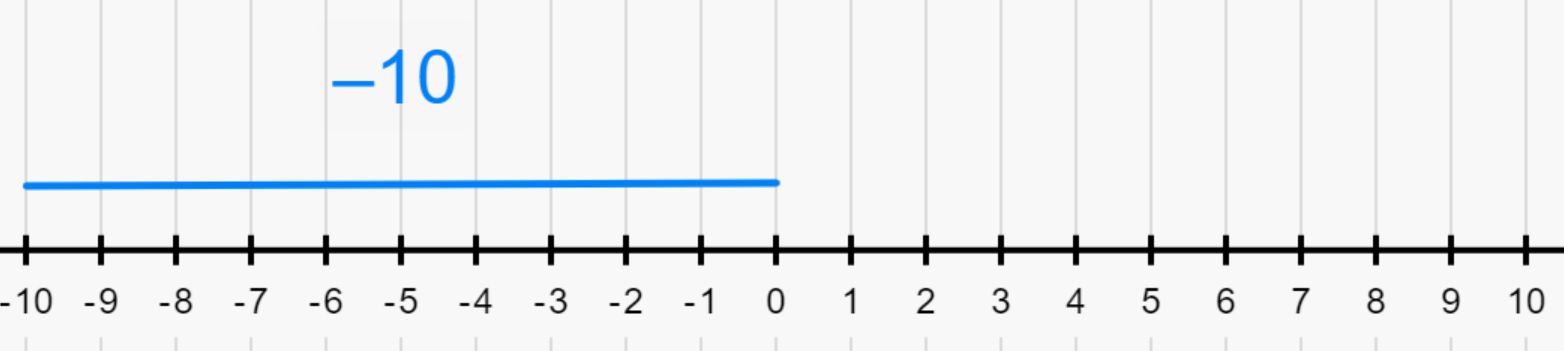–(–10)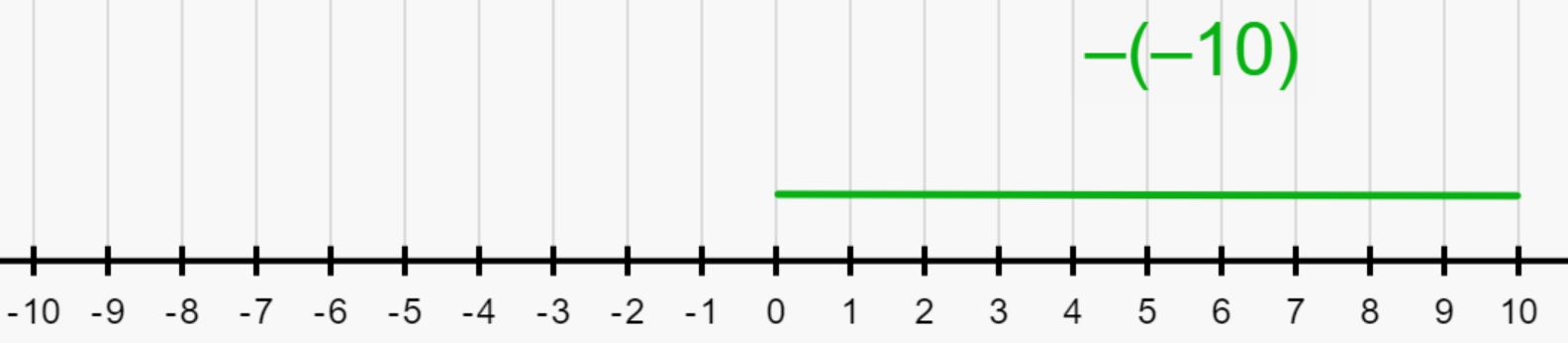As you can see, with the addition of each negative sign the direction of the line flips. That is when we have 10, the number line representation is towards the right ie positive number, when it’s –10, it switches to the left ie negative number, again when another negative sign is added, –(–10), we get back the positive number, 10 and the number line representation switches to the right.